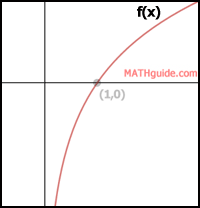Logarithmic Functions: Translations
View the Lesson | MATHguide homepage Updated October 30th, 2019Directions:
Use the graph of f(x) to the right to determine the translation:

g(x) = log5 (x + 3) + 8.

Translation Statement for g(x):

 “The logarithmic function f(x) = log5 (x) must be shifted Select 1 2 3 4 5 6 7 8 units to the Select right left and Select 1 2 3 4 5 6 7 8 units Select up down.”

Characteristics of g(x), to the nearest hundreth:

The domain is (, +∞).
The range is (-∞, +∞).
The asymptote is = .
The x-intercept is (, ).
The point (1,0) on f(x) shifts to (, ) on g(x).